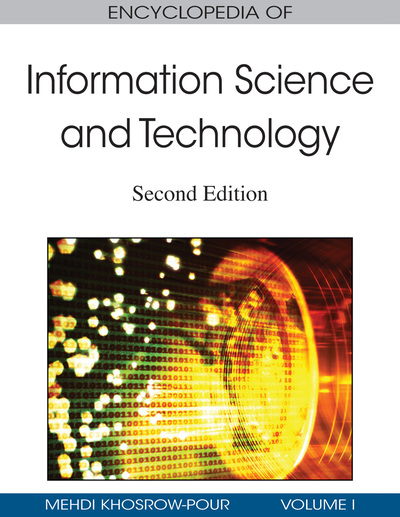# Increasing the Accuracy of Predictive Algorithms: A Review of Ensembles of Classifiers

Sotiris Kotsiantis (University of Patras, Greece & University of Peloponnese, Greece), Dimitris Kanellopoulos (University of Patras, Greece), and Panayotis Pintelas (University of Patras, Greece & University of Peloponnese, Greece)
DOI: 10.4018/978-1-60566-026-4.ch300
Available
\$29.50
No Current Special Offers

## Abstract

In classification learning, the learning scheme is presented with a set of classified examples from which it is expected tone can learn a way of classifying unseen examples (see Table 1). Formally, the problem can be stated as follows: Given training data {(x1, y1)…(xn, yn)}, produce a classifier h: X- >Y that maps an object x ? X to its classification label y ? Y. A large number of classification techniques have been developed based on artificial intelligence (logic-based techniques, perception-based techniques) and statistics (Bayesian networks, instance-based techniques). No single learning algorithm can uniformly outperform other algorithms over all data sets. The concept of combining classifiers is proposed as a new direction for the improvement of the performance of individual machine learning algorithms. Numerous methods have been suggested for the creation of ensembles of classi- fiers (Dietterich, 2000). Although, or perhaps because, many methods of ensemble creation have been proposed, there is as yet no clear picture of which method is best.
Chapter Preview
Top

## Introduction

In classification learning, the learning scheme is presented with a set of classified examples from which it is expected tone can learn a way of classifying unseen examples (see Figure 1).

Formally, the problem can be stated as follows: Given training data {(x1, y1)…(xn, yn)}, produce a classifier h: X->Y that maps an object x є X to its classification label y є Y. A large number of classification techniques have been developed based on artificial intelligence (logic-based techniques, perception-based techniques) and statistics (Bayesian networks, instance-based techniques). No single learning algorithm can uniformly outperform other algorithms over all data sets.

The concept of combining classifiers is proposed as a new direction for the improvement of the performance of individual machine learning algorithms. Numerous methods have been suggested for the creation of ensembles of classifiers (Dietterich, 2000). Although, or perhaps because, many methods of ensemble creation have been proposed, there is as yet no clear picture of which method is best.

Top

## Background

Generally, support vector machines (SVMs; Scholkopf, Burges, & Smola, 1999) and artificial neural networks (ANNs; Mitchell, 1997) tend to perform much better when dealing with multidimensions and continuous features. In contrast, logic-based systems (e.g., decision trees [Murthy, 1998] and rule learners [Furnkranz, 1999]) tend to perform better when dealing with discrete or categorical features. For neural-network models and SVMs, a large sample size is required in order to achieve the maximum prediction accuracy whereas the naive Bayes model (Jensen, 1996) may need a relatively small data set. Most decision-tree algorithms cannot perform well with problems that require diagonal partitioning. The division of the instance space is orthogonal to the axis of one variable and parallel to all other axes. Therefore, the resulting regions after partitioning are all hyperrectangles. The ANNs and the SVMs perform well when multicolinearity is present and a nonlinear relationship exists between the input and output features.

Although training time varies according to the nature of the application task and data set, specialists generally agree on a partial ordering of the major classes of learning algorithms. For instance, lazy learning methods require zero training time because the training instance is simply stored (Aha, 1997). Naive Bayes methods also train very quickly since they require only a single pass on the data either to count frequencies (for discrete variables) or to compute the normal probability density function (for continuous variables under normality assumptions). Univariate decision trees are also reputed to be quite fast—at any rate, several orders of magnitude faster than neural networks and SVMs.

Naive Bayes methods require little storage space during both the training and classification stages: The strict minimum is the memory needed to store the prior and conditional probabilities. The basic k-nearest-neighbor (k-NN) algorithm (Aha, 1997) uses a great deal of storage space for the training phase, and its execution space is at least as big as its training space. On the contrary, for all nonlazy learners, the execution space is usually much smaller than the training space since the resulting classifier is usually a highly condensed summary of the data.

There is general agreement that k-NN is very sensitive to irrelevant features: This characteristic can be explained by the way the algorithm works. In addition, the presence of irrelevant features can make neural-network training very inefficient and even impractical. Logic-based algorithms are all considered very easy to interpret, whereas neural networks and SVMs have notoriously poor interpretability. k-NN is also considered to have very poor interpretability because an unstructured collection of training instances is far from readable, especially if there are many of them.

## Key Terms in this Chapter

Rule Induction: It is the extraction of useful if-then rules from data based on statistical significance.

Majority Voting: The collective prediction is decided by the majority of the votes of the classifiers; that is, the class with the most votes is the final prediction.

Artificial Neural Networks: They are nonlinear predictive models that learn through training and resemble biological neural networks in structure.

Cascade Generalization: It uses the set of classifiers sequentially, at each step performing an extension of the original data by the insertion of new attributes.

Stacking: Stacking integrates the independently computed base classifiers into a higher level classifier: a metaclassifier.

Boosting: It is similar in overall structure to bagging, except that it keeps track of the performance of the learning algorithm and concentrates on instances that have not been correctly learned.

Bagging: Bagging uses different subsets of training data with a single learning method. After the construction of several classifiers, taking a vote of the predictions of each classifier produces the final prediction.

## Complete Chapter List

Search this Book:
Reset